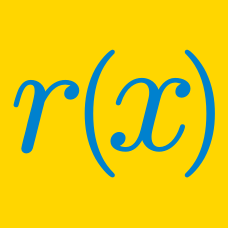Algebra

# Remainder Factor Theorem

If $f(x)=(x-14)(x+1)(x^2+2x+6)$, what is the sum of all real values $x$ satisfying $f(x)=0$?

Consider the polynomial $f(x)=\frac{1}{2}(x-1).$ If $(f(x))^{115}$ leaves a remainder of $ax+b$ upon division by $f\left(x^2\right),$ where $a$ and $b$ are constants, what is the value of $9a+b?$

If the remainder that polynomial $x^3+6x^2+kx-1$ leaves upon division by $x-1$ is $766$, what is the value of constant $k$?

$f(x)$ is a degree 4 polynomial satisfying $f(n) = \frac {1} {n}$ for integers $n =$ 1 to 5. If $f(0) = \frac {a} {b}$, where $a$ and $b$ are coprime positive integers. What is the value of $a + b$?

Find the sum of the values among $3, 6, 9, 12$ that are roots of the polynomial $2x^3-27x^2+63x+162.$

×